# Samacheer Kalvi 6th Maths Solutions Term 1 Chapter 5 Statistics Ex 5.3## Tamilnadu Samacheer Kalvi 6th Maths Solutions Term 1 Chapter 5 Statistics Ex 5.3

Question 1.
Read the given Bar Graph which shows the percentage of marks obtained by Brinda in different subjects in an assessment test.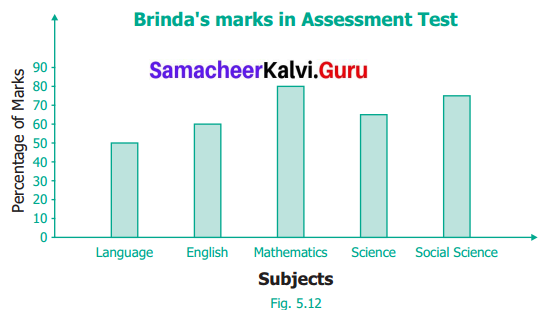Observe the bar graph and answer the following questions.
(i) 1 unit = ___ % of marks on vertical line.
(ii) Brinda has scored maximum marks in _____ subject.
(iii) Brinda has scored minimum marks in ______ subject.
(iv) The percentage of marks scored by Brinda in Science is _____
(v) Brinda scored 60% marks in the subject ______
(vi) Brinda scored 20% more in _______ subject than _____ subject.
Solution:
(i) 10
(ii) Mathematics
(iii) Language
(iv) 65%
(v) English
(vi) Mathematics, English

Question 2.
Chitra has to buy Laddus in order to distribute to her friends as follows: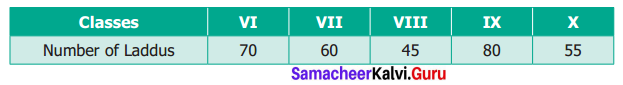Draw a Bar Graph for this data.
Solution: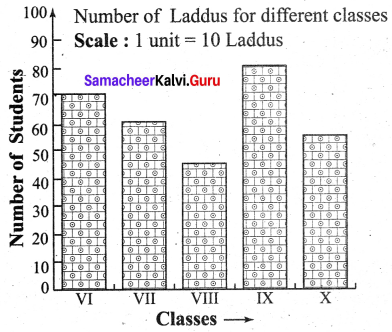Question 3.
The fruits liked by the students of a class are as follows: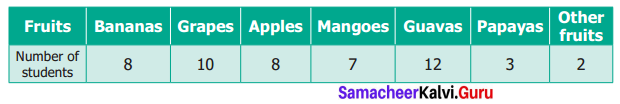Draw a Bar Graph for this data.
Solution:Question 4.
The pictograph below gives the number of absentees on different days of the week in class six. Draw the Bar graph for the same.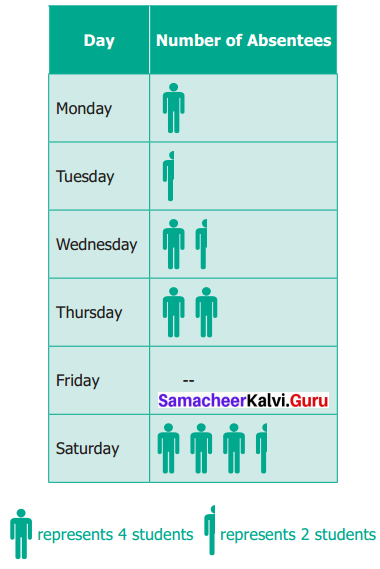Solution: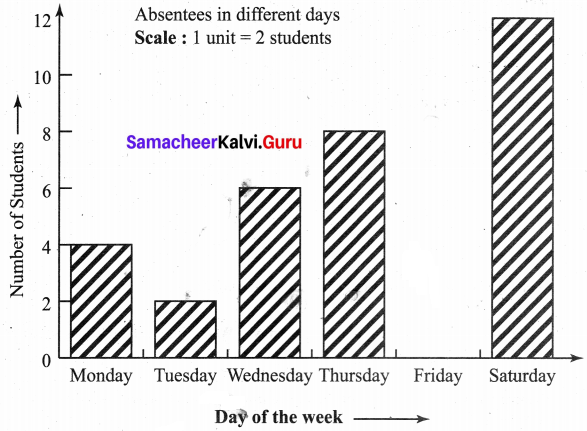Objective Type Questions

Question 5.
A bar graph can be drawn using
(a) Horizontal bars only
(b) Vertical bars only
(c) Both horizontal bars and Vertical bars
(d) Either horizontal bars or vertical bars
Solution:
(d) Either horizontal bars or vertical bars

Question 6.
The spaces between any two bars in a bar graph _____
(a) can be different
(b) are the same
(c) are not the same
(d) all of these
Solution:
(b) are the same